# Create the Mean and Standard Deviation of the Data of a Pandas Series

• Last Updated : 17 Aug, 2020

Standard Deviation is the square root of the Variance. The Standard Deviation denoted by sigma is a measure of the spread of numbers. In pandas, the `std() `function is used to find the standard Deviation of the series.
The mean can be simply defined as the average of numbers. In pandas, the` mean()` function is used to find the mean of the series.

Example 1 : Finding the mean and Standard Deviation of a Pandas Series.

 `# importing the module``import` `pandas as pd`` ` `# creating a series``s ``=` `pd.Series(data ``=` `[``5``, ``9``, ``8``, ``5``, ``7``, ``8``, ``1``, ``2``, ``3``,``                      ``4``, ``5``, ``6``, ``7``, ``8``, ``9``, ``5``, ``3``])`` ` `# displaying the series``print``(s)`

Output :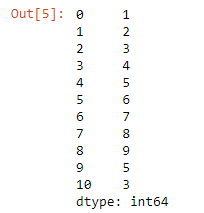Finding the mean of the series using the `mean()` function.

 `# finding the mean``print``(s.mean())`

Output :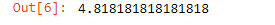Finding the standard deviation of the series using the `std()` function.

 `# finding the Standard deviation``print``(s.std())`

Output :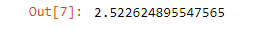Example 2 : Finding the mean and Standard Deviation of a Pandas DataFrame.

 `# importing the module``import` `pandas as pd`` ` `# creating a dataframe ``df ``=` `pd.DataFrame({``'ID'``:[``114``, ``345``, ``157788``, ``5626``],``                   ``'Product'``:[``'shirt'``, ``'trousers'``, ``'tie'``, ``'belt'``],``                   ``'Color'``:[``'White'``, ``'Black'``, ``'Red'``, ``'Brown'``],``                   ``'Discount'``:[``10``, ``10``, ``10``, ``10``]})`` ` `# displaying the DataFrame``print``(df)`

Output :Finding the mean of the DataFrame using the `mean()` function.

 `# finding the mean``print``(df.mean())`

Output :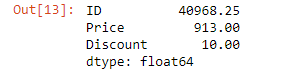Finding the standard deviation of the DataFrame using the `std()` function.

 `# finding the Standard deviation``print``(df.std())`

Output :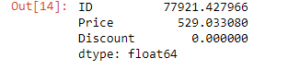My Personal Notes arrow_drop_up